Home > CAAC > Chapter 7 > Lesson 7.1.2 > Problem7-18

7-18.
1. Assume that a baby's length can be determined by the equation l = 23 + 1.5t, where l represents the length of the baby in inches and t represents the age of the baby in months. Homework Help ✎

1. How fast is the baby growing each month? How can you tell?

2. How long was the baby when it was born? How can you tell?

3. How long will the baby be when it is 3 months old?

4. If the baby was born in January, during what month will it be 39.5 inches long?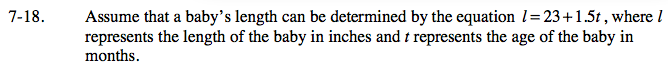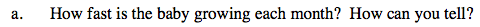The equation is just like the y = mx + b rule, except written in a different order and with different variables.
Knowing this, find how fast the baby is growing each month, or its growth rate.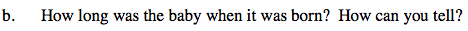Using the hint from part (a), find the length when the baby was born, or its starting value.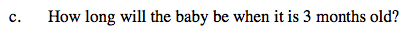Substitute 3 for the time, or the variable t, and solve for the length.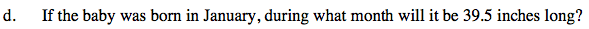First substitute 39.5 for the length and solve for the age of the baby.
Then, add that many months to January to find in what month the baby will be 39.5 inches long.